## 10.6 Noise

In order to write solid textures that describe complex surface appearances, it is helpful to be able to introduce some controlled variation to the process. Consider a wood floor made of individual planks; each plank’s color is likely to be slightly different from the others. Or consider a windswept lake; we might want to have waves of similar amplitude across the entire lake, but we don’t want them to be homogeneous over all parts of the lake (as they might be if they were constructed from a sum of sine waves, for example). Modeling this sort of variation in a texture helps make the final result look more realistic.

One difficulty in developing textures like these is that the renderer evaluates the surface’s texture functions at an irregularly distributed set of points, where each evaluation is completely independent of the others. As such, procedural textures must implicitly define a complex pattern by answering queries about what the pattern’s value is at all of these points. In contrast, the explicit pattern description approach is embodied by the PostScript® language, for example, which describes graphics on a page with a series of drawing commands. One difficulty that the implicit approach introduces is that the texture can’t just call RNG::UniformFloat() at each point at which it is evaluated to introduce randomness: because each point would have a completely different random value from its neighbors, no coherence would be possible in the generated pattern.

An elegant way to address this issue of introducing controlled randomness to procedural textures in graphics is the application of what is known as a noise function. In general, noise functions used in graphics are smoothly varying functions taking , for at least , without obvious repetition. One of the most crucial properties of a practical noise function is that it be band limited with a known maximum frequency. This makes it possible to control the frequency content added to a texture due to the noise function so that frequencies higher than those allowed by the Nyquist limit aren’t introduced.

Many of the noise functions that have been developed are built on the idea of an integer lattice over . First, a value is associated with each integer position in space. Then, given an arbitrary position in space, the eight surrounding lattice values are found. These lattice values are then interpolated to compute the noise value at the particular point. This idea can be generalized or restricted to more or fewer dimensions , where the number of lattice points is . A simple example of this approach is value noise, where pseudo-random numbers between and are associated with each lattice point, and actual noise values are computed with trilinear interpolation or with a more complex spline interpolant, which can give a smoother result by avoiding derivative discontinuities when moving from one lattice cell to another.

For such a noise function, given an integer lattice point, it must be possible to efficiently compute its parameter value in a way that always associates the same value with each lattice point. Because it is infeasible to store values for all possible points, some compact representation is needed. One option is to use a hash function, where the coordinates are hashed and then used to look up parameters from a fixed-size table of precomputed pseudo-random parameter values.

### 10.6.1 Perlin Noise

In pbrt we will implement a noise function introduced by Ken Perlin (1985a, 2002); as such, it is known as Perlin noise. It has a value of zero at all integer lattice points. Its variation comes from gradient vectors at each lattice point that guide the interpolation of a smooth function in between the points (Figure 10.24). This noise function has many of the desired characteristics of a noise function described above, is computationally efficient, and is easy to implement.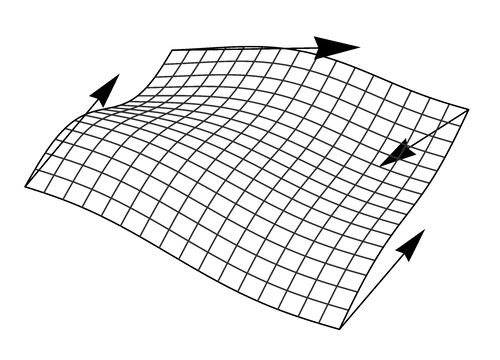Figure 10.25 shows the value of the noise function rendered on a sphere.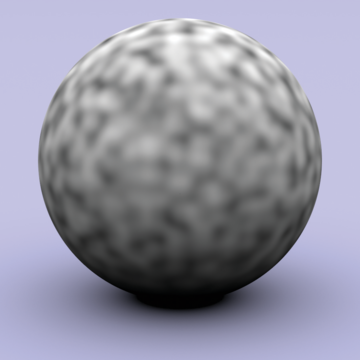<<Texture Method Definitions>>+=
Float Noise(Float x, Float y, Float z) { <<Compute noise cell coordinates and offsets>>
int ix = std::floor(x), iy = std::floor(y), iz = std::floor(z); Float dx = x - ix, dy = y - iy, dz = z - iz;
ix &= NoisePermSize - 1; iy &= NoisePermSize - 1; iz &= NoisePermSize - 1; Float w000 = Grad(ix, iy, iz, dx, dy, dz); Float w100 = Grad(ix+1, iy, iz, dx-1, dy, dz); Float w010 = Grad(ix, iy+1, iz, dx, dy-1, dz); Float w110 = Grad(ix+1, iy+1, iz, dx-1, dy-1, dz); Float w001 = Grad(ix, iy, iz+1, dx, dy, dz-1); Float w101 = Grad(ix+1, iy, iz+1, dx-1, dy, dz-1); Float w011 = Grad(ix, iy+1, iz+1, dx, dy-1, dz-1); Float w111 = Grad(ix+1, iy+1, iz+1, dx-1, dy-1, dz-1);
<<Compute trilinear interpolation of weights>>
Float wx = NoiseWeight(dx), wy = NoiseWeight(dy), wz = NoiseWeight(dz); Float x00 = Lerp(wx, w000, w100); Float x10 = Lerp(wx, w010, w110); Float x01 = Lerp(wx, w001, w101); Float x11 = Lerp(wx, w011, w111); Float y0 = Lerp(wy, x00, x10); Float y1 = Lerp(wy, x01, x11); return Lerp(wz, y0, y1);
}

For convenience, there is also a variant of Noise() that takes a Point3f directly:

<<Texture Method Definitions>>+=
Float Noise(const Point3f &p) { return Noise(p.x, p.y, p.z); }

The implementation first computes the integer coordinates of the cell that contains the given point and the fractional offsets of the point from the lower cell corner:

<<Compute noise cell coordinates and offsets>>=
int ix = std::floor(x), iy = std::floor(y), iz = std::floor(z); Float dx = x - ix, dy = y - iy, dz = z - iz;

It next calls Grad() to get eight weight values, one for each corner of the cell that the point lies inside. Grad() uses the cell indices to index into a table; for efficiency we ensure that all of the indices are within the range of the table here by zeroing any high bits that would put a component past the table’s size. (The table size must be a power of two for this trick to work—otherwise an expensive integer modulus operation would be needed in place of the bitwise “and.”)

ix &= NoisePermSize - 1; iy &= NoisePermSize - 1; iz &= NoisePermSize - 1; Float w000 = Grad(ix, iy, iz, dx, dy, dz); Float w100 = Grad(ix+1, iy, iz, dx-1, dy, dz); Float w010 = Grad(ix, iy+1, iz, dx, dy-1, dz); Float w110 = Grad(ix+1, iy+1, iz, dx-1, dy-1, dz); Float w001 = Grad(ix, iy, iz+1, dx, dy, dz-1); Float w101 = Grad(ix+1, iy, iz+1, dx-1, dy, dz-1); Float w011 = Grad(ix, iy+1, iz+1, dx, dy-1, dz-1); Float w111 = Grad(ix+1, iy+1, iz+1, dx-1, dy-1, dz-1);

Each integer lattice point has a gradient vector associated with it. The influence of the gradient vector for any point inside the cell is obtained by computing the dot product of the vector from the gradient’s corner to the lookup point and the gradient vector (Figure 10.26); this is handled by the Grad() function. Note that the vectors to the corners other than the lower left one can be easily computed incrementally based on that vector.

The gradient vector for a particular integer lattice point is found by indexing into a precomputed table of integer values, NoisePerm. The four low-order bits of the table’s value for the lattice point determine which of 16 gradient vectors is associated with it. In a preprocessing step, this table of size NoisePermSize was filled with numbers from 0 to NoisePermSize-1 and then randomly permuted. These values were then duplicated, making an array of size 2*NoisePermSize that holds the table twice in succession. The second copy of the table makes lookups in the following code slightly more efficient.

Given a particular (ix,iy,iz) lattice point, a series of table lookups gives a value from the random-number table:

NoisePerm[NoisePerm[NoisePerm[ix] + iy] + iz];

By doing three nested permutations in this way, rather than NoisePerm[ix+iy+iz], for example, the final result is more irregular. The first approach doesn’t usually return the same value if ix and iy are interchanged, as the second does. Furthermore, since the table was replicated to be twice the original length, the lookups can be done as described above, eliminating the need for modulus operations in code along the lines of

(NoisePerm[ix] + iy) % NoisePermSize

Given a final value from the permutation table that determines the gradient number, the dot product with the corresponding gradient vector must be computed. However, the gradient vectors do not need to be represented explicitly. All of the gradients use only , , or in their coordinates, so that the dot products reduce to addition of some (possibly negated) components of the vector. The final implementation is the following:

<<Texture Method Definitions>>+=
inline Float Grad(int x, int y, int z, Float dx, Float dy, Float dz) { int h = NoisePerm[NoisePerm[NoisePerm[x] + y] + z]; h &= 15; Float u = h < 8 || h == 12 || h == 13 ? dx : dy; Float v = h < 4 || h == 12 || h == 13 ? dy : dz; return ((h & 1) ? -u : u) + ((h & 2) ? -v : v); }

<<Perlin Noise Data>>=
static constexpr int NoisePermSize = 256; static int NoisePerm[2 * NoisePermSize] = { 151, 160, 137, 91, 90, 15, 131, 13, 201, 95, 96, 53, 194, 233, 7, 225, 140, 36, 103, 30, 69, 142, <<Remainder of the noise permutation table>>
8, 99, 37, 240, 21, 10, 23, 190, 6, 148, 247, 120, 234, 75, 0, 26, 197, 62, 94, 252, 219, 203, 117, 35, 11, 32, 57, 177, 33, 88, 237, 149, 56, 87, 174, 20, 125, 136, 171, 168, 68, 175, 74, 165, 71, 134, 139, 48, 27, 166, 77, 146, 158, 231, 83, 111, 229, 122, 60, 211, 133, 230, 220, 105, 92, 41, 55, 46, 245, 40, 244, 102, 143, 54, 65, 25, 63, 161, 1, 216, 80, 73, 209, 76, 132, 187, 208, 89, 18, 169, 200, 196, 135, 130, 116, 188, 159, 86, 164, 100, 109, 198, 173, 186, 3, 64, 52, 217, 226, 250, 124, 123, 5, 202, 38, 147, 118, 126, 255, 82, 85, 212, 207, 206, 59, 227, 47, 16, 58, 17, 182, 189, 28, 42, 223, 183, 170, 213, 119, 248, 152, 2, 44, 154, 163, 70, 221, 153, 101, 155, 167, 43, 172, 9, 129, 22, 39, 253, 19, 98, 108, 110, 79, 113, 224, 232, 178, 185, 112, 104, 218, 246, 97, 228, 251, 34, 242, 193, 238, 210, 144, 12, 191, 179, 162, 241, 81, 51, 145, 235, 249, 14, 239, 107, 49, 192, 214, 31, 181, 199, 106, 157, 184, 84, 204, 176, 115, 121, 50, 45, 127, 4, 150, 254, 138, 236, 205, 93, 222, 114, 67, 29, 24, 72, 243, 141, 128, 195, 78, 66, 215, 61, 156, 180, 151, 160, 137, 91, 90, 15, 131, 13, 201, 95, 96, 53, 194, 233, 7, 225, 140, 36, 103, 30, 69, 142, 8, 99, 37, 240, 21, 10, 23, 190, 6, 148, 247, 120, 234, 75, 0, 26, 197, 62, 94, 252, 219, 203, 117, 35, 11, 32, 57, 177, 33, 88, 237, 149, 56, 87, 174, 20, 125, 136, 171, 168, 68, 175, 74, 165, 71, 134, 139, 48, 27, 166, 77, 146, 158, 231, 83, 111, 229, 122, 60, 211, 133, 230, 220, 105, 92, 41, 55, 46, 245, 40, 244, 102, 143, 54, 65, 25, 63, 161, 1, 216, 80, 73, 209, 76, 132, 187, 208, 89, 18, 169, 200, 196, 135, 130, 116, 188, 159, 86, 164, 100, 109, 198, 173, 186, 3, 64, 52, 217, 226, 250, 124, 123, 5, 202, 38, 147, 118, 126, 255, 82, 85, 212, 207, 206, 59, 227, 47, 16, 58, 17, 182, 189, 28, 42, 223, 183, 170, 213, 119, 248, 152, 2, 44, 154, 163, 70, 221, 153, 101, 155, 167, 43, 172, 9, 129, 22, 39, 253, 19, 98, 108, 110, 79, 113, 224, 232, 178, 185, 112, 104, 218, 246, 97, 228, 251, 34, 242, 193, 238, 210, 144, 12, 191, 179, 162, 241, 81, 51, 145, 235, 249, 14, 239, 107, 49, 192, 214, 31, 181, 199, 106, 157, 184, 84, 204, 176, 115, 121, 50, 45, 127, 4, 150, 254, 138, 236, 205, 93, 222, 114, 67, 29, 24, 72, 243, 141, 128, 195, 78, 66, 215, 61, 156, 180
};

Given these eight contributions from the gradients, the next step is to trilinearly interpolate between them at the point. Rather than interpolating with dx, dy, and dz directly, though, each of these values is passed through a smoothing function. This ensures that the noise function has first- and second-derivative continuity as lookup points move between lattice cells.

<<Texture Method Definitions>>+=
inline Float NoiseWeight(Float t) { Float t3 = t * t * t; Float t4 = t3 * t; return 6 * t4 * t - 15 * t4 + 10 * t3; }

<<Compute trilinear interpolation of weights>>=
Float wx = NoiseWeight(dx), wy = NoiseWeight(dy), wz = NoiseWeight(dz); Float x00 = Lerp(wx, w000, w100); Float x10 = Lerp(wx, w010, w110); Float x01 = Lerp(wx, w001, w101); Float x11 = Lerp(wx, w011, w111); Float y0 = Lerp(wy, x00, x10); Float y1 = Lerp(wy, x01, x11); return Lerp(wz, y0, y1);

### 10.6.2 Random Polka Dots

A basic use of the noise function is as part of a polka dot texture that divides texture space into rectangular cells (Figure 10.27). Each cell has a 50% chance of having a dot inside of it, and the dots are randomly placed inside their cells. DotsTexture takes the usual 2D mapping function, as well as two Textures, one for the regions of the surface outside of the dots and one for the regions inside. It is defined in the files textures/dots.h and textures/dots.cpp.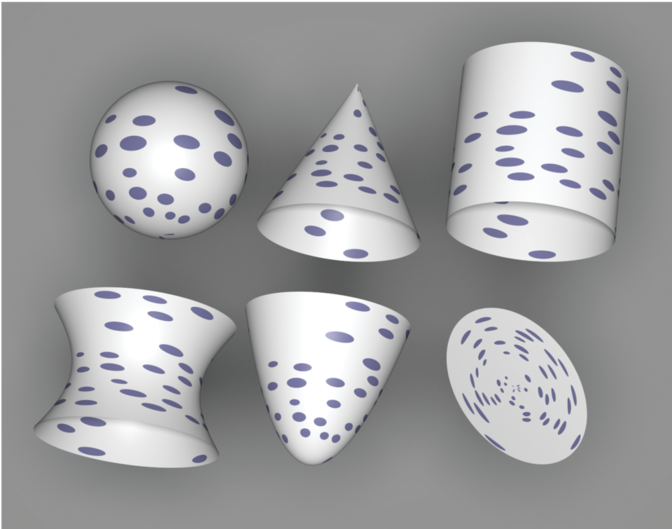<<DotsTexture Declarations>>=
template <typename T> class DotsTexture : public Texture<T> { public: <<DotsTexture Public Methods>>
DotsTexture(std::unique_ptr<TextureMapping2D> mapping, const std::shared_ptr<Texture<T>> &outsideDot, const std::shared_ptr<Texture<T>> &insideDot) : mapping(std::move(mapping)), outsideDot(outsideDot), insideDot(insideDot) { } T Evaluate(const SurfaceInteraction &si) const { <<Compute cell indices for dots>>
Vector2f dstdx, dstdy; Point2f st = mapping->Map(si, &dstdx, &dstdy); int sCell = std::floor(st + .5f), tCell = std::floor(st + .5f);
<<Return insideDot result if point is inside dot>>
if (Noise(sCell + .5f, tCell + .5f) > 0) { Float radius = .35f; Float maxShift = 0.5f - radius; Float sCenter = sCell + maxShift * Noise(sCell + 1.5f, tCell + 2.8f); Float tCenter = tCell + maxShift * Noise(sCell + 4.5f, tCell + 9.8f); Vector2f dst = st - Point2f(sCenter, tCenter); if (dst.LengthSquared() < radius*radius) return insideDot->Evaluate(si); }
return outsideDot->Evaluate(si); }
private: <<DotsTexture Private Data>>
std::unique_ptr<TextureMapping2D> mapping; std::shared_ptr<Texture<T>> outsideDot, insideDot;
};

<<DotsTexture Public Methods>>=
DotsTexture(std::unique_ptr<TextureMapping2D> mapping, const std::shared_ptr<Texture<T>> &outsideDot, const std::shared_ptr<Texture<T>> &insideDot) : mapping(std::move(mapping)), outsideDot(outsideDot), insideDot(insideDot) { }

<<DotsTexture Private Data>>=
std::unique_ptr<TextureMapping2D> mapping; std::shared_ptr<Texture<T>> outsideDot, insideDot;

The evaluation function starts by taking the texture coordinates and computing integer sCell and tCell values, which give the coordinates of the cell they are inside. We will not consider antialiasing of the polka dots texture here; an exercise at the end of the chapter outlines how this might be done.

<<DotsTexture Public Methods>>+=
T Evaluate(const SurfaceInteraction &si) const { <<Compute cell indices for dots>>
Vector2f dstdx, dstdy; Point2f st = mapping->Map(si, &dstdx, &dstdy); int sCell = std::floor(st + .5f), tCell = std::floor(st + .5f);
<<Return insideDot result if point is inside dot>>
if (Noise(sCell + .5f, tCell + .5f) > 0) { Float radius = .35f; Float maxShift = 0.5f - radius; Float sCenter = sCell + maxShift * Noise(sCell + 1.5f, tCell + 2.8f); Float tCenter = tCell + maxShift * Noise(sCell + 4.5f, tCell + 9.8f); Vector2f dst = st - Point2f(sCenter, tCenter); if (dst.LengthSquared() < radius*radius) return insideDot->Evaluate(si); }
return outsideDot->Evaluate(si); }

<<Compute cell indices for dots>>=
Vector2f dstdx, dstdy; Point2f st = mapping->Map(si, &dstdx, &dstdy); int sCell = std::floor(st + .5f), tCell = std::floor(st + .5f);

Once the cell coordinate is known, it’s necessary to decide if there is a polka dot in the cell. Obviously, this computation needs to be consistent so that for each time this routine runs for points in a particular cell it returns the same result. Yet we’d like the result not to be completely regular (e.g., with a dot in every other cell). Noise solves this problem: by evaluating the noise function at a position that is the same for all points inside this cell—(sCell+.5, tCell+.5)—we can compute an irregularly varying but consistent value for each cell. If this value is greater than zero, a dot is placed in the cell.

If there is a dot in the cell, the noise function is used again to randomly shift the center of the dot within the cell. The points at which the noise function is evaluated for the center shift are offset by arbitrary constant amounts, however, so that noise values from different noise cells are used from them, eliminating a possible source of correlation with the noise value used to determine the presence of a dot in the first place. (Note that the dot’s radius must be small enough so that it doesn’t spill over the cell’s boundary after being shifted; in that case, points where the texture was being evaluated would also need to consider the dots based in neighboring cells as potentially affecting their texture value.)

Given the dot center and radius, the texture needs to decide if the coordinates are within the radius of the shifted center. It does this by computing their squared distance to the center and comparing it to the squared radius.

<<Return insideDot result if point is inside dot>>=
if (Noise(sCell + .5f, tCell + .5f) > 0) { Float radius = .35f; Float maxShift = 0.5f - radius; Float sCenter = sCell + maxShift * Noise(sCell + 1.5f, tCell + 2.8f); Float tCenter = tCell + maxShift * Noise(sCell + 4.5f, tCell + 9.8f); Vector2f dst = st - Point2f(sCenter, tCenter); if (dst.LengthSquared() < radius*radius) return insideDot->Evaluate(si); }

### 10.6.3 Noise Idioms and Spectral Synthesis

The fact that noise is a band-limited function means that its frequency content can be adjusted by scaling the domain over which it is evaluated. For example, if Noise(p) has some known frequency content, then the frequency content of Noise(2*p) will be twice as high. This is just like the relationship between the frequency content of and . This technique can be used to create a noise function with a desired rate of variation.

For many applications in procedural texturing, it’s useful to have variation over multiple scales—for example, to add finer variations to the base noise function. One effective way to do this with noise is to compute patterns via spectral synthesis, where a complex function is defined by a sum of contributions from another function :

for a set of weight values and parameter scale values . If the base function has a well-defined frequency content (e.g., is a sine or cosine function or a noise function), then each term also has a well-defined frequency content as described earlier. Because each term of the sum is weighted by a weight value , the result is a sum of contributions of various frequencies, with different frequency ranges weighted differently.

Typically, the scales are chosen in a geometric progression such that and the weights are . The result is that as higher frequency variation is added to the function, it has relatively less influence on the overall shape of . Each additional term is called an octave of noise, since it has twice the frequency content of the previous one. When this scheme is used with Perlin noise, the result is often referred to as fractional Brownian motion (fBm), after a particular type of random process that varies in a similar manner.

Fractional Brownian motion is a useful building block for procedural textures because it gives a function with more complex variation than plain noise, while still being easy to compute and still having well-defined frequency content. The utility function FBm() implements the fractional Brownian motion function. Figure 10.28 shows two graphs of it.

In addition to the point at which to evaluate the function and the function’s partial derivatives at that point, the function takes an omega parameter, which ranges from zero to one and affects the smoothness of the pattern by controlling the falloff of contributions at higher frequencies (values around work well), and maxOctaves, which gives the maximum number of octaves of noise that should be used in computing the sum.

<<Texture Method Definitions>>+=
Float FBm(const Point3f &p, const Vector3f &dpdx, const Vector3f &dpdy, Float omega, int maxOctaves) { <<Compute number of octaves for antialiased FBm>>
Float len2 = std::max(dpdx.LengthSquared(), dpdy.LengthSquared()); Float n = Clamp(-1 - .5f * Log2(len2), 0, maxOctaves); int nInt = std::floor(n);
<<Compute sum of octaves of noise for FBm>>
Float sum = 0, lambda = 1, o = 1; for (int i = 0; i < nInt; ++i) { sum += o * Noise(lambda * p); lambda *= 1.99f; o *= omega; } Float nPartial = n - nInt; sum += o * SmoothStep(.3f, .7f, nPartial) * Noise(lambda * p);
return sum; }

The implementation here uses a technique called clamping to antialias the fBm function. The idea is that when we are computing a value based on a sum of components, each with known frequency content, we should stop adding in components that would have frequencies beyond the Nyquist limit and instead add their average values to the sum. Because the average value of Noise() is zero, all that needs to be done is to compute the number of octaves such that none of the terms has excessively high frequencies and not evaluate the noise function for any higher octaves.

Noise() (and thus the first term of as well) has a maximum frequency content of roughly . Each subsequent term represents a doubling of frequency content. Therefore, we would like to find the appropriate number of terms such that if the sampling rate in noise space is , then

which ensures that there are frequencies right up to the Nyquist frequency but not past it. Equivalently,

where we’ve used the identity to write the last expression in a more convenient form for the following.

The squared sampling rate can be computed with one over the maximum of the squared length of the differentials and , which we’ll denote by . We can turn this inversion into a negation of the logarithm, and equivalently write this as:

<<Compute number of octaves for antialiased FBm>>=
Float len2 = std::max(dpdx.LengthSquared(), dpdy.LengthSquared()); Float n = Clamp(-1 - .5f * Log2(len2), 0, maxOctaves); int nInt = std::floor(n);

Finally, the integral number of octaves up to the Nyquist limit are added together and the last octave is faded in, according to the fractional part of n. This ensures that successive octaves of noise fade in gradually, rather than appearing abruptly, which can cause visually noticeable artifacts at the transitions. The implementation here actually increases the frequency between octaves by 1.99, rather than by a factor of 2, in order to reduce the impact of the fact that the noise function is zero at integer lattice points. This breaks up that regularity across sums of octaves of noise, which can also lead to subtle visual artifacts.

<<Compute sum of octaves of noise for FBm>>=
Float sum = 0, lambda = 1, o = 1; for (int i = 0; i < nInt; ++i) { sum += o * Noise(lambda * p); lambda *= 1.99f; o *= omega; } Float nPartial = n - nInt; sum += o * SmoothStep(.3f, .7f, nPartial) * Noise(lambda * p);

The SmoothStep() function takes a minimum and maximum value and a point at which to evaluate a smooth interpolating function. If the point is below the minimum zero is returned, and if it’s above the maximum one is returned. Otherwise, it smoothly interpolates between zero and one using a cubic Hermite spline.

<<Texture Inline Functions>>=
inline Float SmoothStep(Float min, Float max, Float value) { Float v = Clamp((value - min) / (max - min), 0, 1); return v * v * (-2 * v + 3); }

Closely related to the FBm() function is the Turbulence() function. It also computes a sum of terms of the noise function but takes the absolute value of each one:

Taking the absolute value introduces first-derivative discontinuities in the synthesized function, and thus the turbulence function has infinite frequency content. Nevertheless, the visual characteristics of this function can be quite useful for procedural textures. Figure 10.29 shows two graphs of the turbulence function.

The Turbulence() implementation here tries to antialias itself in the same way that FBm() did. As described earlier, however, the first-derivative discontinuities in the function introduce infinitely high-frequency content, so these efforts can’t hope to be perfectly successful. The Turbulence() antialiasing here at least eliminates some of the worst of the artifacts; otherwise, increasing the pixel sampling rate is the best recourse. In practice, this function doesn’t alias too terribly when used in procedural textures, particularly compared to the aliasing from infinitely high frequencies from geometric and shadow edges.

<<Texture Method Definitions>>+=
Float Turbulence(const Point3f &p, const Vector3f &dpdx, const Vector3f &dpdy, Float omega, int maxOctaves) { <<Compute number of octaves for antialiased FBm>>
Float len2 = std::max(dpdx.LengthSquared(), dpdy.LengthSquared()); Float n = Clamp(-1 - .5f * Log2(len2), 0, maxOctaves); int nInt = std::floor(n);
<<Compute sum of octaves of noise for turbulence>>
Float sum = 0, lambda = 1, o = 1; for (int i = 0; i < nInt; ++i) { sum += o * std::abs(Noise(lambda * p)); lambda *= 1.99f; o *= omega; }
<<Account for contributions of clamped octaves in turbulence>>
Float nPartial = n - nInt; sum += o * Lerp(SmoothStep(.3f, .7f, nPartial), 0.2, std::abs(Noise(lambda * p))); for (int i = nInt; i < maxOctaves; ++i) { sum += o * 0.2f; o *= omega; }
return sum; }

<<Compute sum of octaves of noise for turbulence>>=
Float sum = 0, lambda = 1, o = 1; for (int i = 0; i < nInt; ++i) { sum += o * std::abs(Noise(lambda * p)); lambda *= 1.99f; o *= omega; }

The average value of the absolute value of the noise function is roughly 0.2; this value should be added to the sum for the octaves where the noise function’s estimated frequency would be higher than the sampling rate.

<<Account for contributions of clamped octaves in turbulence>>=
Float nPartial = n - nInt; sum += o * Lerp(SmoothStep(.3f, .7f, nPartial), 0.2, std::abs(Noise(lambda * p))); for (int i = nInt; i < maxOctaves; ++i) { sum += o * 0.2f; o *= omega; }

### 10.6.4 Bumpy and Wrinkled Textures

The fBm and turbulence functions are particularly useful as a source of random variation for bump mapping. The FBmTexture is a Float-valued texture that uses FBm() to compute offsets, and WrinkledTexture uses Turbulence() to do so. They are demonstrated in Figures 10.30 and 10.31 and are implemented in textures/fbm.h, textures/fbm.cpp, textures/wrinkled.h, and textures/wrinkled.cpp.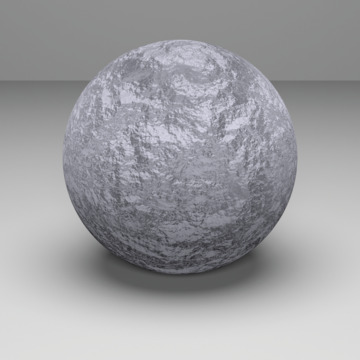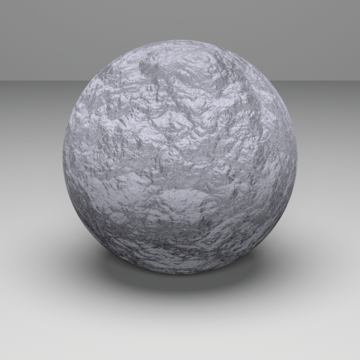<<FBmTexture Declarations>>=
template <typename T> class FBmTexture : public Texture<T> { public: <<FBmTexture Public Methods>>
FBmTexture(std::unique_ptr<TextureMapping3D> mapping, int octaves, Float omega) : mapping(std::move(mapping)), omega(omega), octaves(octaves) { } T Evaluate(const SurfaceInteraction &si) const { Vector3f dpdx, dpdy; Point3f P = mapping->Map(si, &dpdx, &dpdy); return FBm(P, dpdx, dpdy, omega, octaves); }
private: std::unique_ptr<TextureMapping3D> mapping; const Float omega; const int octaves; };

<<FBmTexture Public Methods>>=
FBmTexture(std::unique_ptr<TextureMapping3D> mapping, int octaves, Float omega) : mapping(std::move(mapping)), omega(omega), octaves(octaves) { }

<<FBmTexture Public Methods>>+=
T Evaluate(const SurfaceInteraction &si) const { Vector3f dpdx, dpdy; Point3f P = mapping->Map(si, &dpdx, &dpdy); return FBm(P, dpdx, dpdy, omega, octaves); }

The implementation of WrinkledTexture is almost identical to FBmTexture, save for a call to Turbulence() instead of FBm(). As such, it isn’t included here.

### 10.6.5 Windy Waves

Application of fBm can give a reasonably convincing representation of waves (Ebert et al. 2003). Figures 1.11 and 4.1 use this texture for the water in those scenes. This Texture is based on two observations. First, across the surface of a wind-swept lake (for example), some areas are relatively smooth and some are more choppy; this effect comes from the natural variation of the wind’s strength from area to area. Second, the overall form of individual waves on the surface can be described well by the fBm-based wave pattern scaled by the wind strength. This texture is implemented in textures/windy.h and textures/windy.cpp.

<<WindyTexture Declarations>>=
template <typename T> class WindyTexture : public Texture<T> { public: <<WindyTexture Public Methods>>
WindyTexture(std::unique_ptr<TextureMapping3D> mapping) : mapping(std::move(mapping)) { } T Evaluate(const SurfaceInteraction &si) const { Vector3f dpdx, dpdy; Point3f P = mapping->Map(si, &dpdx, &dpdy); Float windStrength = FBm(.1f * P, .1f * dpdx, .1f * dpdy, .5, 3); Float waveHeight = FBm(P, dpdx, dpdy, .5, 6); return std::abs(windStrength) * waveHeight; }
private: std::unique_ptr<TextureMapping3D> mapping; };

<<WindyTexture Public Methods>>=
WindyTexture(std::unique_ptr<TextureMapping3D> mapping) : mapping(std::move(mapping)) { }

The evaluation function uses two calls to the FBm() function. The first scales down the point P by a factor of 10; as a result, the first call to FBm() returns relatively low-frequency variation over the surface of the object being shaded. This value is used to determine the local strength of the wind. The second call determines the amplitude of the wave at the particular point, independent of the amount of wind there. The product of these two values gives the actual wave offset for the particular location.

<<WindyTexture Public Methods>>+=
T Evaluate(const SurfaceInteraction &si) const { Vector3f dpdx, dpdy; Point3f P = mapping->Map(si, &dpdx, &dpdy); Float windStrength = FBm(.1f * P, .1f * dpdx, .1f * dpdy, .5, 3); Float waveHeight = FBm(P, dpdx, dpdy, .5, 6); return std::abs(windStrength) * waveHeight; }

### 10.6.6 Marble

Another classic use of the noise function is to perturb texture coordinates before using another texture or lookup table. For example, a facsimile of marble can be made by modeling the marble material as a series of layered strata and then using noise to perturb the coordinate used for finding a value among the strata. The MarbleTexture in this section implements this approach. Figure 10.32 illustrates the idea behind this texture. On the left, the layers of marble are indexed directly using the coordinate of the point on the sphere. On the right, fBm has been used to perturb the value, introducing variation. This texture is implemented in textures/marble.h and textures/marble.cpp.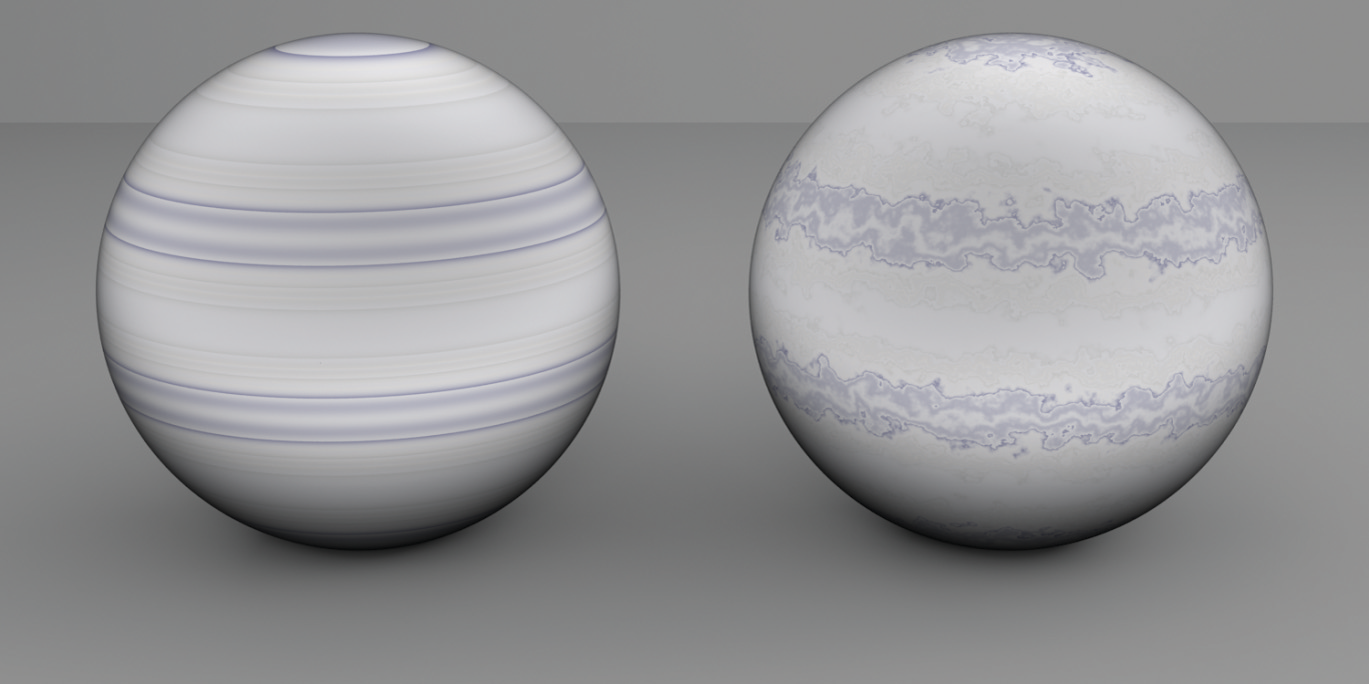<<MarbleTexture Declarations>>=
class MarbleTexture : public Texture<Spectrum> { public: <<MarbleTexture Public Methods>>
MarbleTexture(std::unique_ptr<TextureMapping3D> mapping, int octaves, Float omega, Float scale, Float variation) : mapping(std::move(mapping)), octaves(octaves), omega(omega), scale(scale), variation(variation) { } Spectrum Evaluate(const SurfaceInteraction &si) const { Vector3f dpdx, dpdy; Point3f p = mapping->Map(si, &dpdx, &dpdy); p *= scale; Float marble = p.y + variation * FBm(p, scale * dpdx, scale * dpdy, omega, octaves); Float t = .5f + .5f * std::sin(marble); <<Evaluate marble spline at t>>
static Float c[] = { { .58f, .58f, .6f }, { .58f, .58f, .6f }, { .58f, .58f, .6f }, { .5f, .5f, .5f }, { .6f, .59f, .58f }, { .58f, .58f, .6f }, { .58f, .58f, .6f }, {.2f, .2f, .33f }, { .58f, .58f, .6f }, }; #define NC sizeof(c) / sizeof(c) #define NSEG (NC-3) int first = std::floor(t * NSEG); t = (t * NSEG - first); Spectrum c0 = Spectrum::FromRGB(c[first]); Spectrum c1 = Spectrum::FromRGB(c[first+1]); Spectrum c2 = Spectrum::FromRGB(c[first+2]); Spectrum c3 = Spectrum::FromRGB(c[first+3]); // Bezier spline evaluated with de Castilejau's algorithm Spectrum s0 = (1.f - t) * c0 + t * c1; Spectrum s1 = (1.f - t) * c1 + t * c2; Spectrum s2 = (1.f - t) * c2 + t * c3; s0 = (1.f - t) * s0 + t * s1; s1 = (1.f - t) * s1 + t * s2; // Extra scale of 1.5 to increase variation among colors return 1.5f * ((1.f - t) * s0 + t * s1);
}
private: <<MarbleTexture Private Data>>
std::unique_ptr<TextureMapping3D> mapping; const int octaves; const Float omega, scale, variation;
};

The texture takes the usual set of parameters to control the FBm() function that will be used to perturb the lookup coordinate. The variation parameter modulates the magnitude of the perturbation.

<<MarbleTexture Public Methods>>=
MarbleTexture(std::unique_ptr<TextureMapping3D> mapping, int octaves, Float omega, Float scale, Float variation) : mapping(std::move(mapping)), octaves(octaves), omega(omega), scale(scale), variation(variation) { }

<<MarbleTexture Private Data>>=
std::unique_ptr<TextureMapping3D> mapping; const int octaves; const Float omega, scale, variation;

An offset into the marble layers is computed by adding the variation to the point’s component and using the sine function to remap its value into the range . The <<Evaluate marble spline at t>> fragment uses the value as the evaluation point for a cubic spline through a series of colors that are similar to those of real marble.

<<MarbleTexture Public Methods>>+=
Spectrum Evaluate(const SurfaceInteraction &si) const { Vector3f dpdx, dpdy; Point3f p = mapping->Map(si, &dpdx, &dpdy); p *= scale; Float marble = p.y + variation * FBm(p, scale * dpdx, scale * dpdy, omega, octaves); Float t = .5f + .5f * std::sin(marble); <<Evaluate marble spline at t>>
static Float c[] = { { .58f, .58f, .6f }, { .58f, .58f, .6f }, { .58f, .58f, .6f }, { .5f, .5f, .5f }, { .6f, .59f, .58f }, { .58f, .58f, .6f }, { .58f, .58f, .6f }, {.2f, .2f, .33f }, { .58f, .58f, .6f }, }; #define NC sizeof(c) / sizeof(c) #define NSEG (NC-3) int first = std::floor(t * NSEG); t = (t * NSEG - first); Spectrum c0 = Spectrum::FromRGB(c[first]); Spectrum c1 = Spectrum::FromRGB(c[first+1]); Spectrum c2 = Spectrum::FromRGB(c[first+2]); Spectrum c3 = Spectrum::FromRGB(c[first+3]); // Bezier spline evaluated with de Castilejau's algorithm Spectrum s0 = (1.f - t) * c0 + t * c1; Spectrum s1 = (1.f - t) * c1 + t * c2; Spectrum s2 = (1.f - t) * c2 + t * c3; s0 = (1.f - t) * s0 + t * s1; s1 = (1.f - t) * s1 + t * s2; // Extra scale of 1.5 to increase variation among colors return 1.5f * ((1.f - t) * s0 + t * s1);
}